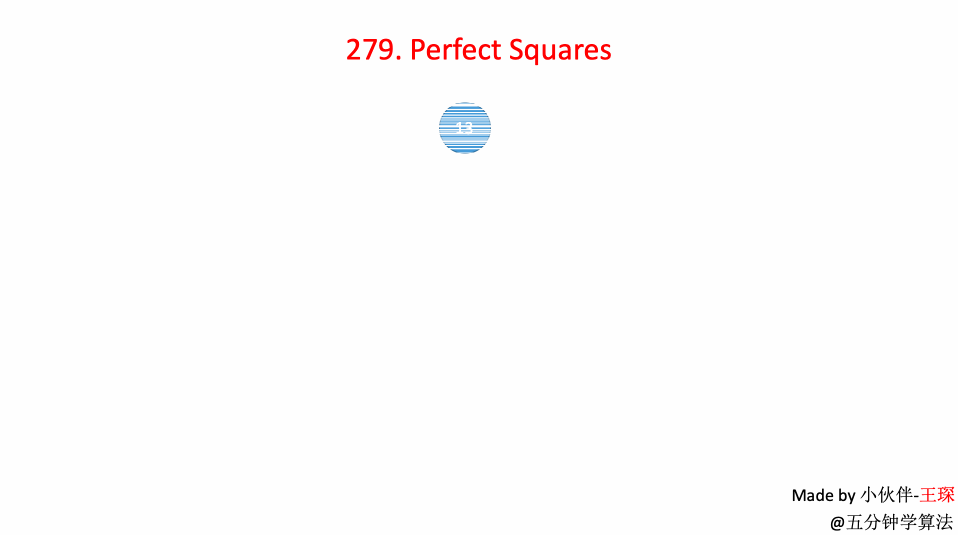# LeetCode 第 279 号问题：完全平方数

### 题目描述

``````输入: n = 12

``````

``````输入: n = 13

``````

### 题目解析

``````public int numSquares(int n) {
while (n % 4 == 0){
n /= 4;
}
if ( n % 8 == 7){
return 4;
}
int a = 0;
while ( (a * a) <= n){
int b = (int)Math.pow((n - a * a),0.5);
if(a * a + b * b == n) {
//如果可以 在这里返回
if(a != 0 && b != 0) {
return 2;
} else{
return 1;
}
}
a++;
}
return 3;
}
``````

### 动画描述### 代码实现

``````import java.util.LinkedList;
import javafx.util.Pair;
class Solution {
public int numSquares(int n) {
if(n == 0)
return 0;

LinkedList<Pair<Integer, Integer>> queue = new LinkedList<Pair<Integer, Integer>>();
queue.addLast(new Pair<Integer, Integer>(n, 0));

boolean[] visited = new boolean[n+1];
visited[n] = true;

while(!queue.isEmpty()){
Pair<Integer, Integer> front = queue.removeFirst();
int num = front.getKey();
int step = front.getValue();

if(num == 0)
return step;

for(int i = 1 ; num - i*i >= 0 ; i ++){
int a = num - i*i;
if(!visited[a]){
if(a == 0) return step + 1;
queue.addLast(new Pair(num - i * i, step + 1));
visited[num - i * i] = true;
}
}
}
return 0;
}
}
``````